Next: The Nyquist frequency Up: Discrete Fourier transform Previous: Discrete Fourier transform

# FT AS AN INVERTIBLE MATRIX

A Fourier sum may be written(1)
where the complex value Z is related to the real frequencyby.This Fourier sum is a way of building a continuous function offrom discrete signal values bt in the time domain. In this chapter we will study the computational tricks associated with specifying both time and frequency domains by a set of points. Begin with an example of a signal that is nonzero at four successive instants, ( b0, b1, b2, b3). The transform is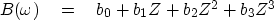(2)
The evaluation of this polynomial can be organized as a matrix times a vector, such as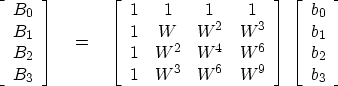(3)
Observe that the top row of the matrix evaluates the polynomial at Z=1, a point where also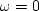.The second row evaluates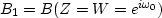,whereis some base frequency. The third row evaluates the Fourier transform for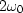,and the bottom row for.The matrix could have more than four rows for more frequencies and more columns for more time points. I have made the matrix square in order to show you next how we can find the inverse matrix. The size of the matrix in (3) is N=4. If we choose the base frequencyand hence W correctly, the inverse matrix will be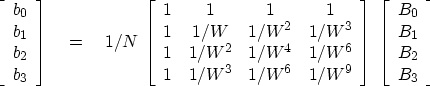(4)
Multiplying the matrix of (4) with that of (3), we first see that the diagonals are +1 as desired. To have the off diagonals vanish, we need various sums, such as 1+W +W2+W3 and 1+W2+W4+W6, to vanish. Every element (W6, for example, or 1/W9) is a unit vector in the complex plane. In order for the sums of the unit vectors to vanish, we must ensure that the vectors pull symmetrically away from the origin. A uniform distribution of directions meets this requirement. In other words, W should be the N-th root of unity, i.e.,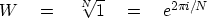(5)

The lowest frequency is zero, corresponding to the top row of (3). The next-to-the-lowest frequency we find by setting W in (5) to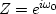.So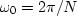; and for (4) to be inverse to (3), the frequencies required are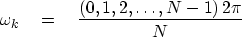(6)Next: The Nyquist frequency Up: Discrete Fourier transform Previous: Discrete Fourier transform
Stanford Exploration Project
10/21/1998